# SAT Math Multiple Choice Question 925: Answer and Explanation

### Test Information

Question: 925

9. A commercial airline has calculated that the approximate fuel mileage for its 600-passengerairplane is 0.2 miles per gallon when the plane travels at an average speed of 500miles per hour. Flight 818's fuel tank has 42,000 gallons of fuel at the beginningof an international flight. If the plane travels at an average speed of 500 milesper hour, which of the following functions f models the number of gallons of fuel remaining in the tank t hours after the flight begins?

• A.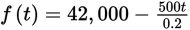• B.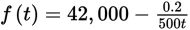• C.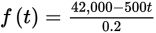• D.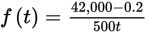Getting to the Answer: The airplane is traveling at an average speed of 500 miles per hour, and the plane's fuel mileage is 0.2 miles per gallon. You can calculate the number of gallons of fuel used each hour using the factor-label method: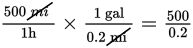gal/h. This means that, in t hours, the plane uses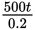gallons of fuel. The plane's tank has 42,000 gallons of fuel at the beginning of the flight, so the amount of fuel left after t hours is 42, 000 -. This makes (A) the correct answer.